# pyaibox.misc package

## pyaibox.misc.bounding_box module

pyaibox.misc.bounding_box.fmt_bbox(bboxes, fmtstr='LTRB2CHW')

Formats bounding boxes

Warning

The height and width are computed by $$y_{\rm max} - y_{\rm min}$$ and $$x_{\rm max} - x_{\rm min}$$.

Parameters
• bboxes (list or numpy array) – The bounding boxes to be converted, all bboxes have the same mode.

• fmtstr (str, optional) –

• 'LTRB2TLBR': LeftTopRightBottom (xmin, ymin, xmax, ymax) –> TopLeftBottomRight (ymin, xmin, ymax, xmax)

• 'TLBR2LTRB': TopLeftBottomRight (ymin, xmin, ymax, xmax) –> LeftTopRightBottom (xmin, ymin, xmax, ymax)

• 'CWH2CHW': CenterWidthHeight (x, y, w, h) –> CenterHeightWidth (y, x, h, w)

• 'CHW2CWH': CenterHeightWidth (y, x, h, w) –> CenterWidthHeight (x, y, w, h)

• 'LTRB2CWH': LeftTopRightBottom (xmin, ymin, xmax, ymax) –> CenterWidthHeight (x, y, w, h)

• 'LTRB2CHW': LeftTopRightBottom (xmin, ymin, xmax, ymax) –> CenterHeightWidth (y, x, h, w)

• 'TLBR2CWH': TopLeftBottomRight (ymin, xmin, ymax, xmax) –> CenterWidthHeight (x, y, w, h)

• 'TLBR2CHW': TopLeftBottomRight (ymin, xmin, ymax, xmax) –> CenterHeightWidth (y, x, h, w)

• 'CWH2LTRB': CenterWidthHeight (x, y, w, h) –> LeftTopRightBottom (xmin, ymin, xmax, ymax)

• 'CWH2TLBR': CenterWidthHeight (x, y, w, h) –> TopLeftBottomRight (ymin, xmin, ymax, xmax)

• 'CHW2LTRB': CenterHeightWidth (y, x, h, w) –> LeftTopRightBottom (xmin, ymin, xmax, ymax)

• 'CHW2TLBR': CenterHeightWidth (y, x, h, w) –> TopLeftBottomRight (ymin, xmin, ymax, xmax)

• 'LRTB2LTRB': LeftRightTopBottom (xmin, xmax, ymin, ymax) –> LeftTopRightBottom (xmin, ymin, xmax, ymax)

• 'LRTB2TLBR': LeftRightTopBottom (xmin, xmax, ymin, ymax) –> TopLeftBottomRight (ymin, xmin, ymax, xmax)

• 'LTRB2LRTB': LeftTopRightBottom (xmin, ymin, xmax, ymax) –> LeftRightTopBottom (xmin, xmax, ymin, ymax)

• 'LRTB2CWH': LeftRightTopBottom (xmin, xmax, ymin, ymax) –> CenterWidthHeight (x, y, w, h)

• 'LRTB2CHW': LeftRightTopBottom (xmin, xmax, ymin, ymax) –> CenterHeightWidth (y, x, h, w)

• 'CWH2LRTB': CenterWidthHeight (x, y, w, h) –> LeftRightTopBottom (xmin, xmax, ymin, ymax)

• 'CHW2LRTB': CenterHeightWidth (y, x, h, w) –> LeftRightTopBottom (xmin, xmax, ymin, ymax)

Returns

• list or numpy array – The formated bounding boxes.

pyaibox.misc.bounding_box.plot_bbox(bboxes, labels=None, scores=None, edgecolors=None, linewidths=1, fontdict=None, textpos='TopCenter', offset=None, ax=None)

Plots bounding boxes with scores and labels

Parameters
• bboxes (list or numpy array) – The bounding boxes, in LeftTopRightBottom mode, which means (xmin, ymin, xmax, ymax)

• labels (list or None, optional) – The labels, can be a list of class id or class name. If None, won’t show labels.

• scores (list or None, optional) – The scores, can be a list of float numbers. If None, won’t show labels.

• edgecolors (None, optional) – The edgecolors for bounding boxes.

• linewidths (int, optional) – The linewidths for bounding boxes.

• fontdict (None, optional) – The fontdict for labels and scores.

• textpos (str, optional) – The position for text (labels and scores).

• offset (None, optional) – The offset for text (labels and scores).

• ax (None, optional) – The ax handle, If None, auto generated.

Returns

• ax – The ax handle

Example

Plot bounding boxes with scores and labels on an image.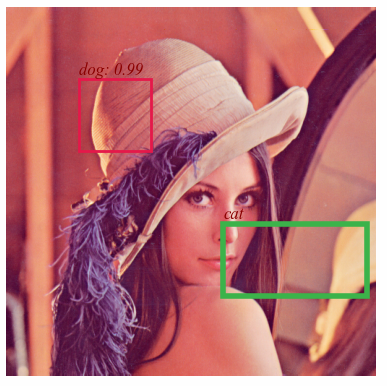The results shown in the above figure can be obtained by the following codes.

import pyaibox as pl
import matplotlib.pyplot as plt

bboxes = [[100, 100, 200, 200], [300, 300, 400, 500]]
labels = ['dog', 'cat']
scores = [0.987, None]
edgecolors = [list(pb.DISTINCT_COLORS_RGB_NORM.values()), None]
edgecolors = list(pb.DISTINCT_COLORS_RGB_NORM.values())[0:2]
linewidths = [2, 4]

fontdict = {'family': 'Times New Roman',
'style': 'italic',
'weight': 'normal',
'color': 'darkred',
'size': 12,
}

fig = plt.figure()
ax.imshow(x)

pb.plot_bbox(bboxes, labels=labels, scores=scores, edgecolors=edgecolors, linewidths=linewidths, fontdict=fontdict, textpos='TopLeft', ax=ax)
plt.axis('off')
plt.show()


## pyaibox.misc.draw_shapes module

pyaibox.misc.draw_shapes.draw_rectangle(x, rects, edgecolors=[[255, 0, 0]], linewidths=, fillcolors=[None], axes=(-3, -2))

Draw rectangles in a tensor

Parameters
• x (numpy array) – The input with any size.

• rects (list or tuple) – The coordinates of the rectangles [[lefttop, rightbottom]].

• edgecolors (list, optional) – The color of edge.

• linewidths (int, optional) – The linewidths of edge.

• fillcolors (int, optional) – The color for filling.

• axes (int, optional) – The axes for drawing the rect (default [(-3, -2)]).

Returns

• x (numpy array) – Output image array with rectangle shapes.

• see fmt_bbox()

Example

Draw rectangles in an figure, and return the result image array.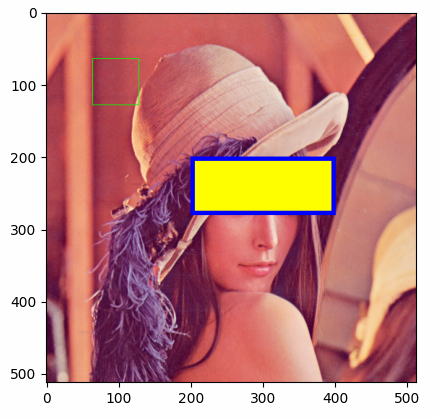The results shown in the above figure can be obtained by the following codes.

import pyaibox as pl
import matplotlib.pyplot as plt

print(pb.__version__)

print(x.shape)

# rects, edgecolors, fillcolors, linewidths = [[0, 0, 511, 511]], [None], [[0, 255, 0]], 
# rects, edgecolors, fillcolors, linewidths = [[0, 0, 511, 511]], [[255, 0, 0]], [None], 
# rects, edgecolors, fillcolors, linewidths = [[0, 0, 511, 511]], [[255, 0, 0]], [[0, 255, 0]], 
rects, edgecolors, fillcolors, linewidths = [[64, 64, 128, 128], [200, 200, 280, 400]], [[0, 255, 0], [0, 0, 255]], [None, [255, 255, 0]], [1, 6]

y = pb.draw_rectangle(x, rects, edgecolors=edgecolors, linewidths=linewidths, fillcolors=fillcolors, axes=[(0, 1)])

pb.imsave('out.png', y)
plt.figure()
plt.imshow(y)
plt.show()


## pyaibox.misc.mapping_operation module

pyaibox.misc.mapping_operation.mapping(X, drange=(0.0, 255.0), mode='amplitude', method='2Sigma', odtype='auto')

convert to image

Convert data to image data $$\bm X$$ with dynamic range $$d=[min, max]$$.

Parameters
• X (numpy array) – data to be converted

• drange (tuple, optional) – dynamic range (the default is (0., 255.))

• mode (str, optional) – data mode in X, 'amplitude' (default) or 'power'.

• method (str, optional) – converting method, surpported values are '1Sigma', '2Sigma', '3Sigma' (the default is ‘2Sigma’, which means two-sigma mapping)

• odtype (str, None..., optional) – output data type, supportted are 'auto' (auto infer, default), or numpy.ndarray’s dtype string. If the type of odtype is not string, the output data type is 'th.float32'.

Returns

Y – converted image data

Return type

numpy array

## pyaibox.misc.noising module

pyaibox.misc.noising.awgn(sig, snrv=30, pmode='db', power='measured', seed=None)

AWGN Add white Gaussian noise to a signal.

AWGN Add white Gaussian noise to a signal like matlab.

Y = AWGN(X,snrv) adds white Gaussian noise to X. The snrv is in dB. The power of X is assumed to be 0 dBW. If X is complex, then AWGN adds complex noise.

Parameters
• sig (tensor) – Signal that will be noised.

• snrv (float, optional) – Signal Noise Ratio (the default is 30)

• pmode (str, optional) – Power mode 'linear', 'db' (the default is ‘db’)

• power (float, str, optional) – the power of signal or the method for computing power (the default is ‘measured’, which is sigPower = np.sum(np.abs(sig) ** 2) / np.numel(sig))

• seed (int, optional) – Seed for random number generator. (the default is None, which means different each time)

Returns

noised data

Return type

tensor

Raises
pyaibox.misc.noising.awgns(x, snrv, **kwargs)

adds white gaussian noise to signal

Parameters
• x (tensor) – The pure signal data.

• snrv (int or float) – The signal-to-noise ratio value in dB.

• caxis (None or int, optional) – If x is complex-valued but represented in real format, caxis or cdim should be specified. If not, it’s set to None, which means x is real-valued or complex-valued in complex format.

• keepcaxis (int or None, optional) – keep the complex dimension?

• axis (int or None, optional) – Specifies the dimensions for adding noise, if not specified, it’s set to None, which means all the dimensions.

• seed (int or None, optional) – Specifies the seed for generating random noise, if not specified, it’s set to None.

• extra (bool, optional) – If True, noise will also be returned.

Returns

• y (tensor) – The SNRs.

Examples

import torch as th
import pyaibox as pb

pb.setseed(2020)
x = np.random.randn(5, 2, 3, 4)
x = pb.r2c(x, caxis=1)  # 5, 3, 4
y, n = awgns(x, 30, axis=(1, 2), seed=2022, extra=True)
snrv = pb.snr(y, n, axis=(1, 2))
print(snrv, 'complex-valued in complex-format')

pb.setseed(2020)
x = np.random.randn(5, 2, 3, 4)
y, n = awgns(x, 30, caxis=1, keepcaxis=False, axis=(1, 2), seed=2022, extra=True)
snrv = pb.snr(y, n, caxis=1, keepcaxis=False, axis=(1, 2))
print(snrv, 'complex-valued in real-format')

pb.setseed(2020)
x = np.random.randn(5, 2, 3, 4)
y, n = awgns(x, 30, caxis=None, axis=(1, 2, 3), seed=2022, extra=True)
snrv = pb.snr(y, n, caxis=None, axis=(1, 2, 3))
print(snrv, 'real-valued in real-format')

pb.setseed(2020)
x = np.random.randn(5, 2, 3, 4)
y, n = awgns2(x, 30, caxis=1, axis=(2, 3), seed=2022, extra=True)
snrv = pb.snr(y, n, caxis=1, axis=(1, 2), keepcaxis=False)
print(snrv, 'real-valued in real-format, multi-channel')

# ---output
[29.97444457 30.06965181 29.95413251 29.99284633 29.96209985] complex-valued in complex-format
[29.97444457 30.06965181 29.95413251 29.99284633 29.96209985] complex-valued in real-format
[29.99399902 30.04417082 30.05313719 29.86315167 29.9423689 ] real-valued in real-format
[29.97424739 30.07329346 29.95404992 29.98695481 29.94601035] real-valued in real-format, multi-channel

pyaibox.misc.noising.awgns2(x, snrv, **kwargs)

adds white gaussian noise to multi-channel signal

Parameters
• x (tensor) – The pure real-valued multi-channel signal data.

• snrv (int or float) – The signal-to-noise ratio value in dB.

• caxis (None or int, optional) – Specifies the channel dimension. If not specified, x will be treated as single-channel signal.

• axis (int or None, optional) – Specifies the dimensions for adding noise, if not specified, it’s set to None, which means all the dimensions.

• seed (int or None, optional) – Specifies the seed for generating random noise, if not specified, it’s set to None.

• extra (bool, optional) – If True, noise will also be returned.

Returns

• y (tensor) – The SNRs.

Examples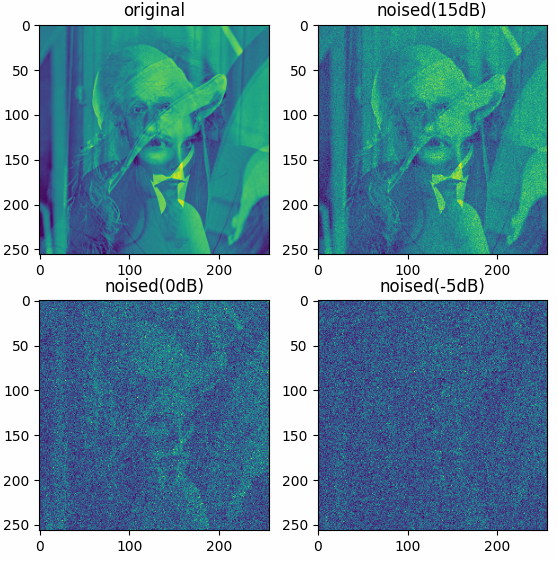The results shown in the above figure can be obtained by the following codes.

datafolder = pb.data_path('optical')

x = xr + 1j * xi
x = pb.c2r(x, caxis=-1)
print(x.shape)

xnp15, np15 = pb.awgns2(x, snrv=15, caxis=-1, axis=(0, 1), extra=True)
xn0, n0 = pb.awgns2(x, snrv=0, caxis=-1, axis=(0, 1), extra=True)
xnn5, nn5 = pb.awgns2(x, snrv=-5, caxis=-1, axis=(0, 1), extra=True)

print(pb.snr(x, np15, caxis=-1, axis=(0, 1)))
print(pb.snr(x, n0, caxis=-1, axis=(0, 1)))
print(pb.snr(x, nn5, caxis=-1, axis=(0, 1)))

x = pb.abs(x, caxis=-1)
xnp15 = pb.abs(xnp15, caxis=-1)
xn0 = pb.abs(xn0, caxis=-1)
xnn5 = pb.abs(xnn5, caxis=-1)

plt = pb.imshow([x, xnp15, xn0, xnn5], titles=['original', 'noised(15dB)', 'noised(0dB)', 'noised(-5dB)'])
plt.show()

pyaibox.misc.noising.imnoise(x, noise='awgn', snrv=30, fmt='chnllast', seed=None)

Add noise to each channel of the image.

Parameters
• x (tensor) – image aray

• noise (str, optional) – noise type (the default is ‘awgn’, which means white gaussian noise, using awgn())

• snrv (float, optional) – Signal-to-noise ratio (the default is 30, which [default_description])

• peak (None, str or float) – Peak value in input, if None, auto detected (default), if 'maxv', use the maximum value as peak value.

• fmt (str or None, optional) – for color image, fmt should be specified with 'chnllast' or 'chnlfirst', for gray image, fmt should be setted to None.

Returns

Return type

tensor

Examples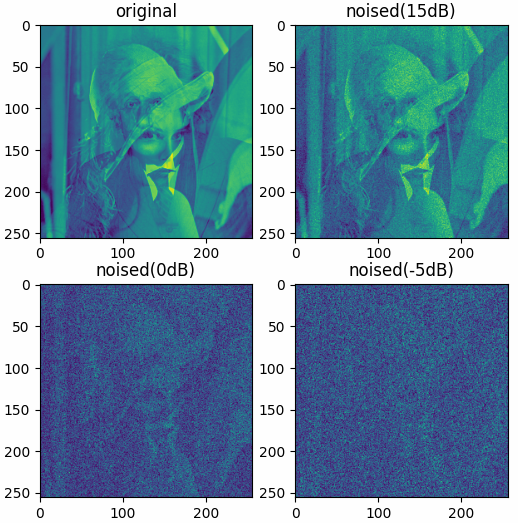The results shown in the above figure can be obtained by the following codes.

datafolder = pb.data_path('optical')

x = xr + 1j * xi

xnp15 = pb.imnoise(x, 'awgn', snrv=15)
xn0 = pb.imnoise(x, 'awgn', snrv=0)
xnn5 = pb.imnoise(x, 'awgn', snrv=-5)

x = pb.abs(x, caxis=None)
xnp15 = pb.abs(xnp15, caxis=None)
xn0 = pb.abs(xn0, caxis=None)
xnn5 = pb.abs(xnn5, caxis=None)

plt = pb.imshow([x, xnp15, xn0, xnn5], titles=['original', 'noised(15dB)', 'noised(0dB)', 'noised(-5dB)'])
plt.show()

datafolder = pb.data_path('optical')

x = xr + 1j * xi
x = pb.c2r(x, caxis=-1)
print(x.shape, x.max())

xnp15 = pb.imnoise(x, 'awgn', snrv=15)
xn0 = pb.imnoise(x, 'awgn', snrv=0)
xnn5 = pb.imnoise(x, 'awgn', snrv=-5)

x = pb.abs(x, caxis=-1)
xnp15 = pb.abs(xnp15, caxis=-1)
xn0 = pb.abs(xn0, caxis=-1)
xnn5 = pb.abs(xnn5, caxis=-1)

plt = pb.imshow([x, xnp15, xn0, xnn5], titles=['original', 'noised(15dB)', 'noised(0dB)', 'noised(-5dB)'])
plt.show()

pyaibox.misc.noising.wgn(shape, power, pmode='dbw', dtype='real', seed=None)

WGN Generates white Gaussian noise.

WGN Generates white Gaussian noise like matlab.

Y = WGN((M,N),P) generates an M-by-N matrix of white Gaussian noise. P specifies the power of the output noise in dBW. The unit of measure for the output of the wgn function is Volts. For power calculations, it is assumed that there is a load of 1 Ohm.

Parameters
• shape (tuple) – Shape of noising matrix

• power (float) – P specifies the power of the output noise in dBW.

• pmode (str, optional) – Power mode of the output noise (the default is ‘dbw’)

• dtype (str, optional) – data type, real or complex (the default is ‘real’, which means real-valued)

• seed (int, optional) – Seed for random number generator. (the default is None, which means different each time)

Returns

Matrix of white Gaussian noise (real or complex).

Return type

tensor

## pyaibox.misc.sampling module

pyaibox.misc.sampling.dnsampling(x, ratio=1.0, axis=-1, smode='uniform', omode='discard', seed=None, extra=False)

down-sampling a tensor

Parameters
• x (ndarray) – The input tensor.

• ratio (float, optional) – Downsampling ratio.

• axis (int, optional) – Downsampling axis (default -1).

• smode (str, optional) – Downsampling mode: 'uniform', 'random', 'random2'.

• omode (str, optional) – output mode: 'discard' for discarding, 'zero' for zero filling.

• seed (int or None, optional) – seed for numpy’s random.

• extra (bool, optional) – If True, also return sampling mask.

Returns

Description

Return type

(ndarray)

Raises
pyaibox.misc.sampling.patch2tensor(p, size=(256, 256), axis=(1, 2), mode='nfirst')

merge patch to a tensor

Parameters
• p (tensor) – A tensor of patches.

• size (tuple, optional) – Merged tensor size in the dimension (the default is (256, 256)).

• axis (tuple, optional) – Merged axis of patch (the default is (1, 2))

• mode (str, optional) – Patch mode 'nfirst' or 'nlast' (the default is ‘nfirst’, which means the first dimension is the number of patches)

Returns

Merged tensor.

Return type

ndarray

pyaibox.misc.sampling.read_samples(datafiles, keys=[['SI', 'ca', 'cr']], nsamples=, groups=, mode='sequentially', axis=0, parts=None, seed=None)

Parameters
• datafiles (list) – list of path strings

• keys (list, optional) – data keys to be read

• nsamples (list, optional) – number of samples for each data file

• groups (list, optional) – number of groups in each data file

• mode (str, optional) – sampling mode for all datafiles

• axis (int, optional) – sampling axis for all datafiles

• parts (None, optional) – number of parts (split samples into some parts)

• seed (None, optional) – the seed for random stream

Returns

samples

Return type

tensor

Raises

ValueErrornsamples should be large enough

pyaibox.misc.sampling.sample_tensor(x, n, axis=0, groups=1, mode='sequentially', seed=None, extra=False)

sample a tensor

Sample a tensor sequentially/uniformly/randomly.

Parameters
• x (ndarray) – a numpy or torch tensor to be sampled

• n (int) – sample number

• axis (int, optional) – the axis to be sampled (the default is 0)

• groups (int, optional) – number of groups in this tensor (the default is 1)

• mode (str, optional) –

• 'sequentially': evenly spaced (default)

• 'uniformly': [0, int(n/groups)]

• 'randomly': randomly selected, non-returned sampling

• seed (None or int, optional) – only work for 'randomly' mode (the default is None)

• extra (bool, optional) – If True, also return the selected indexes, the default is False.

Returns

Sampled numpy or torch tensor. idx (list): Sampled indexes, if extra is True, this will also be returned.

Return type

y (ndarray)

Example

setseed(2020, 'numpy')

x = np.randint(1000, (20, 3, 4))
y1, idx1 = sample_tensor(x, 10, axis=0, groups=2, mode='sequentially', extra=True)
y2, idx2 = sample_tensor(x, 10, axis=0, groups=2, mode='uniformly', extra=True)
y3, idx3 = sample_tensor(x, 10, axis=0, groups=2, mode='randomly', extra=True)

print(x.shape)
print(y1.shape)
print(y2.shape)
print(y3.shape)
print(idx1)
print(idx2)
print(idx3)

the outputs are as follows:

torch.Size([20, 3, 4])
torch.Size([10, 3, 4])
torch.Size([10, 3, 4])
torch.Size([10, 3, 4])
[0, 1, 2, 3, 4, 10, 11, 12, 13, 14]
[0, 2, 4, 6, 8, 10, 12, 14, 16, 18]
[3, 1, 5, 8, 7, 17, 18, 13, 16, 10]

Raises

ValueError – The tensor does not has enough samples.

pyaibox.misc.sampling.shuffle_tensor(x, axis=0, groups=1, mode='inter', seed=None, extra=False)

shuffle a tensor

Shuffle a tensor randomly.

Parameters
• x (ndarray) – A numpy or torch tensor to be shuffled.

• axis (int, optional) – The axis to be shuffled (default 0)

• groups (number, optional) – The number of groups in this tensor (default 1)

• mode (str, optional) –

• 'inter': between groups (default)

• 'intra': within group

• 'whole': the whole

• seed (None or number, optional) – random seed (the default is None)

• extra (bool, optional) – If True, also returns the shuffle indexes, the default is False.

Returns

Shuffled numpy or torch tensor. idx (list): Shuffled indexes, if extra is True, this will also be returned.

Return type

y (ndarray)

Example

Shuffle a tensor randomly with different modes ('intra', 'inter', 'whole').

setseed(2020, 'numpy')

x = np.randint(1000, (20, 3, 4))
y1, idx1 = shuffle_tensor(x, axis=0, groups=4, mode='intra', extra=True)
y2, idx2 = shuffle_tensor(x, axis=0, groups=4, mode='inter', extra=True)
y3, idx3 = shuffle_tensor(x, axis=0, groups=4, mode='whole', extra=True)

print(x.shape)
print(y1.shape)
print(y2.shape)
print(y3.shape)
print(idx1)
print(idx2)
print(idx3)

the outputs are as follows:

torch.Size([20, 3, 4])
torch.Size([20, 3, 4])
torch.Size([20, 3, 4])
torch.Size([20, 3, 4])
[1, 0, 3, 4, 2, 8, 6, 5, 9, 7, 13, 11, 12, 14, 10, 18, 15, 17, 16, 19]
[0, 1, 2, 3, 4, 10, 11, 12, 13, 14, 5, 6, 7, 8, 9, 15, 16, 17, 18, 19]
[1, 13, 12, 5, 19, 9, 11, 6, 4, 16, 17, 3, 8, 18, 7, 10, 15, 0, 14, 2]

pyaibox.misc.sampling.slidegrid(start, stop, step, shake=0, n=None)

generates sliding grid indexes

Generates n sliding grid indexes from start to stop with step size step.

Parameters
• start (int or list) – start sampling point

• stop (int or list) – stop sampling point

• step (int or list) – sampling stepsize

• shake (float) – the shake rate, if shake is 0, no shake, (default), if positive, add a positive shake, if negative, add a negative.

• n (int or None) – the number of samples (default None, int((stop0 - start0) / step0) * int((stop1 - start1) / step1)…).

Returns

for multi-dimension, return a 2-d tensor, for 1-dimension, return a 1d-tensor.

Raises

TypeError – The number of samples should be an integer or None.

see randperm(), randgrid().

pyaibox.misc.sampling.split_tensor(x, ratios=[0.7, 0.2, 0.1], axis=0, shuffle=False, seed=None, extra=False)

split a tensor

split a tensor into some parts.

Parameters
• x (ndarray) – A numpy array or torch tensor.

• ratios (list, optional) – Split ratios (the default is [0.7, 0.2, 0.05])

• axis (int, optional) – Split axis (the default is 0)

• shuffle (bool, optional) – Whether shuffle (the default is False)

• seed (int, optional) – Shuffule seed (the default is None)

• extra (bool, optional) – If True, also return the split indexes, the default is False.

Returns

Splitted ndarrays.

Return type

(list of ndarray)

pyaibox.misc.sampling.tensor2patch(x, n=None, size=(256, 256), axis=(0, 1), start=(0, 0), stop=(None, None), step=(1, 1), shake=(0, 0), mode='slidegrid', seed=None)

sample patch from a tensor

Sample some patches from a tensor, tensor and patch can be any size.

Parameters
• x (ndarray) – A tensor to be sampled.

• n (int, optional) – The number of pactches, the default is None, auto computed, equals to the number of blocks with specified step

• size (tuple or int, optional) – The size of patch (the default is (256, 256))

• axis (tuple or int, optional) – The sampling axis (the default is (0, 1))

• start (tuple or int, optional) – Start sampling index for each axis (the default is (0, 0))

• stop (tuple or int, optional) – Stopp sampling index for each axis. (the default is (None, None), which [default_description])

• step (tuple or int, optional) – Sampling stepsize for each axis (the default is (1, 1), which [default_description])

• shake (tuple or int or float, optional) – float for shake rate, int for shake points (the default is (0, 0), which means no shake)

• mode (str, optional) – Sampling mode, 'slidegrid', 'randgrid', 'randperm' (the default is ‘slidegrid’)

• seed (int, optional) – Random seed. (the default is None, which means no seed.)

Returns

A tensor of sampled patches.

Return type

(ndarray)

Example

Sample patches from a tensor with different mode (randperm, randgrid, slidegrid), and then reform these patches into an image.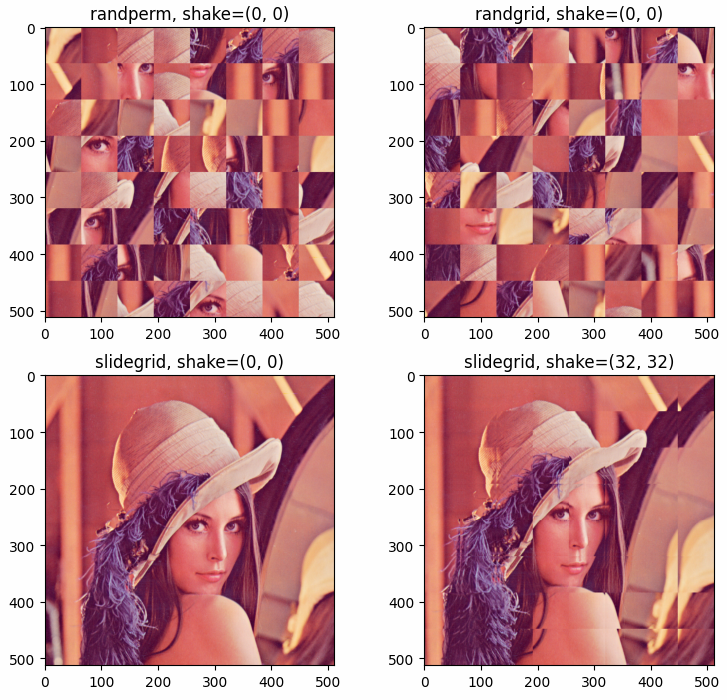The results shown in the above figure can be obtained by the following codes.

import math
import numpy as np
import pyaibox as pl
import matplotlib.pyplot as plt

filename = '../../data/images/Lotus512.png'
filename = '../../data/images/LenaRGB512.tif'

xshape = x.shape
xshape = xshape[:2]

n, size = 64, (64, 64)

y1 = pb.tensor2patch(x, n=n, size=size, axis=(0, 1), step=(1, 1), shake=(0, 0), mode='randperm', seed=2020)
y2 = pb.tensor2patch(x, n=n, size=size, axis=(0, 1), step=(64, 64), shake=(0, 0), mode='randgrid', seed=2020)
y3 = pb.tensor2patch(x, n=n, size=size, axis=(0, 1), step=(64, 64), shake=(0, 0), mode='slidegrid', seed=2020)
y4 = pb.tensor2patch(x, n=n, size=size, axis=(0, 1), step=(64, 64), shake=(32, 32), mode='slidegrid', seed=2020)

print(y1.shape, y2.shape, y3.shape, y4.shape)

Y1 = pb.patch2tensor(y1, size=xshape, axis=(1, 2), mode='nfirst')
Y2 = pb.patch2tensor(y2, size=xshape, axis=(1, 2), mode='nfirst')
Y3 = pb.patch2tensor(y3, size=xshape, axis=(1, 2), mode='nfirst')
Y4 = pb.patch2tensor(y4, size=xshape, axis=(1, 2), mode='nfirst')

plt.figure()
plt.subplot(221)
plt.imshow(Y1)
plt.title('randperm, shake=(0, 0)')
plt.subplot(222)
plt.imshow(Y2)
plt.title('randgrid, shake=(0, 0)')
plt.subplot(223)
plt.imshow(Y3)
plt.title('slidegrid, shake=(0, 0)')
plt.subplot(224)
plt.imshow(Y4)
plt.title('slidegrid, shake=(32, 32)')
plt.show()


## pyaibox.misc.transform module

pyaibox.misc.transform.ct2rt(x, axis=0)

Converts a complex-valued tensor to a real-valued tensor

Converts a complex-valued tensor $${\bf x}$$ to a real-valued tensor with FFT and conjugate symmetry.

Parameters
• x (Tensor) – The input tensor $${\bf x}$$.

• axis (int) – The axis for excuting FFT.

Returns

Examples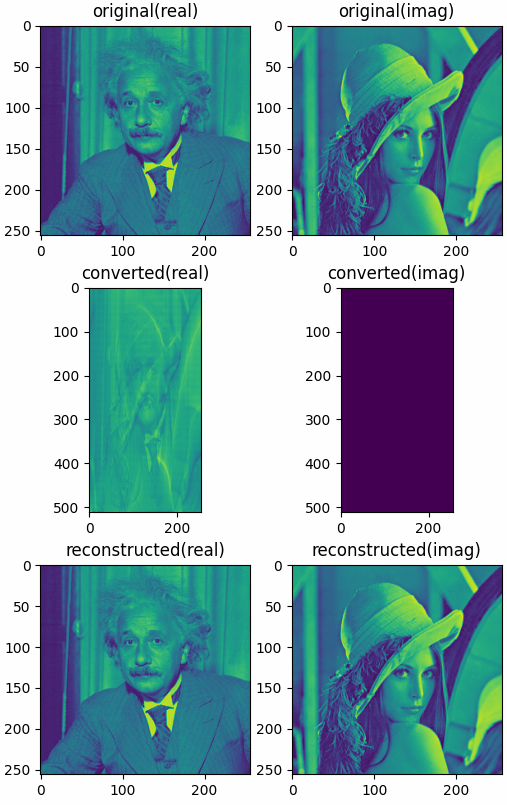The results shown in the above figure can be obtained by the following codes.

import numpy as np
import pyaibox as pb

datafolder = pb.data_path('optical')

x = xr + 1j * xi

y = pb.ct2rt(x, axis=0)
z = pb.rt2ct(y, axis=0)

print(x.shape, y.shape, z.shape)
print(x.dtype, y.dtype, z.dtype)

print(np.min(np.abs(x)), np.max(np.abs(x)))
print(np.min(np.abs(y)), np.max(np.abs(y)))
print(np.min(np.abs(z)), np.max(np.abs(z)))

plt = pb.imshow([x.real, x.imag, y.real, y.imag, z.real, z.imag], nrows=3, ncols=2,
titles=['original(real)', 'original(imag)', 'converted(real)',
'converted(imag)', 'reconstructed(real)', 'reconstructed(imag)'])
plt.show()

pyaibox.misc.transform.db20(x)

compute dB

Parameters

x (float or array) – linear value

Returns

the dB value of x.

Return type

float or array

pyaibox.misc.transform.quantization(X, idrange=None, odrange=[0, 31], odtype='auto', extra=False)

Quantize data.

${\bm X} \in [a, b] \rightarrow y \in [c, d]$
${\bm Y} = \lfloor (d-c) (X-a) / (b-a) + c \rfloor.$
Parameters
• X (ndarray) – The data to be quantized with shape $${\bm X} \in {\mathbb R}^{N_a×N_r}$$, or $${\bm X} \in {\mathbb C}^{N_a×N_r}$$.

• idrange (tuple, list, optional) – Specifies the range of data. Default $$[{\rm min}(X), {\rm max}(X)]$$.

• odrange (tuple, list, optional) – Specifies the range of data after beening quantized. Default [0, 31].

• odtype (str or None, optional) – output data type, supportted are 'auto' (auto infer, default), or numpy ndarray’s dtype string. If the type of odtype is not string(such as None), the type of output data is the same with input.

• extra (bool) – If True, also return idrange and odrange.

Returns

Quantized data ndarray, if the input is complex, will return a ndarray with shape $${\bm Y} \in {\mathbb R}^{N_a×N_r×2}$$. idrange, odrange (list or tuple): If extra is true, also be returned

Return type

(ndarray)

Raises

Exceptionidrange and odrange should be (tulpe) or (list)

pyaibox.misc.transform.rt2ct(y, axis=0)

Converts a real-valued tensor to a complex-valued tensor

Converts a real-valued tensor $${\bf y}$$ to a complex-valued tensor with FFT and conjugate symmetry.

Parameters
• y (Tensor) – The input tensor $${\bf y}$$.

• axis (int) – The axis for excuting FFT.

Returns

pyaibox.misc.transform.scale(X, st=[0, 1], sf=None, istrunc=True, extra=False)

Scale data.

$x \in [a, b] \rightarrow y \in [c, d]$
$y = (d-c)*(x-a) / (b-a) + c.$
Parameters
• X (ndarray) – The data to be scaled.

• st (tuple, list, optional) – Specifies the range of data after beening scaled. Default [0, 1].

• sf (tuple, list, optional) – Specifies the range of data. Default [min(X), max(X)].

• istrunc (bool) – Specifies wether to truncate the data to [a, b], For example, If sf == [a, b] and ‘istrunc’ is true, then X[X < a] == a and X[X > b] == b.

• extra (bool) – If True, also return st and sf.

Returns

Scaled data ndarray. st, sf (list or tuple): If extra is true, also be returned

Return type

out (ndarray)

Raises

Exception – Description

pyaibox.misc.transform.standardization(X, mean=None, std=None, axis=None, extra=False)
$\bar{X} = \frac{X-\mu}{\sigma}$
Parameters
• X (ndarray) – data to be normalized,

• mean (list or None, optional) – mean value (the default is None, which means auto computed)

• std (list or None, optional) – standard deviation (the default is None, which means auto computed)

• axis (list, tuple or int, optional) – specify the axis for computing mean and standard deviation (the default is None, which means all elements)

• extra (bool, optional) – if True, also return the mean and std (the default is False, which means just return the standardized data)

Returns

Standardized/Normalized ndarray.

Return type

(ndarray)